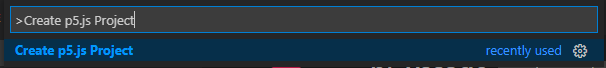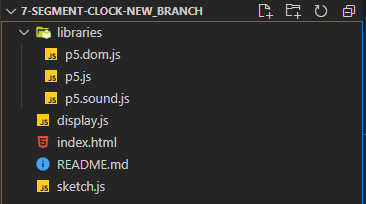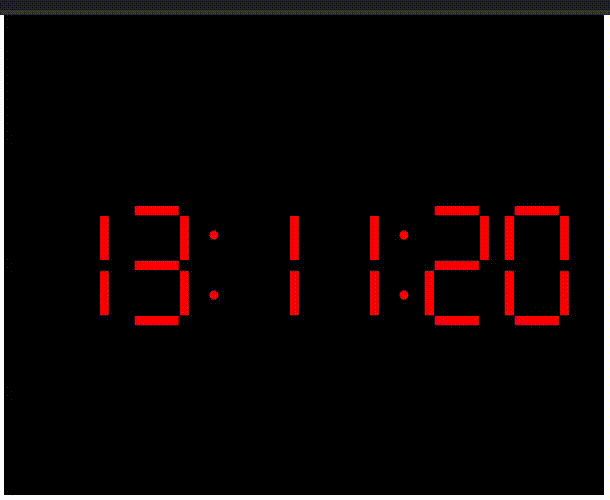# How to create seven segment clock using p5.js library?

• Difficulty Level : Hard
• Last Updated : 31 Mar, 2021

The seven-segment display is a form of an electronic display device for displaying decimal numerals that is an alternative to the more complex dot matrix displays.

7 segment display clocks each segment is a single led and all the 7 LEDs connected together with a common pin that may be a common positive or a common negative and arranged in a specific style. A typical 7 segment display has 10 pins arranged in the top and bottoms each of the middle pins is common pin. Seven-segment displays are widely used in digital clocks, electronic meters, basic calculators, and other electronic devices that display numerical information.

Hey geek! The constant emerging technologies in the world of web development always keeps the excitement for this subject through the roof. But before you tackle the big projects, we suggest you start by learning the basics. Kickstart your web development journey by learning JS concepts with our JavaScript Course. Now at it's lowest price ever!

p5.js is a JavaScript library for creative coding, with a focus on making coding accessible and inclusive for artists, designers, educators, beginners, and anyone else! p5.js is free and open-source because we believe software, and the tools to learn it, should be accessible to everyone.

Using the metaphor of a sketch, p5.js has a full set of drawing functionality. However, you’re not limited to your drawing canvas. You can think of your whole browser page as your sketch, including HTML5 objects for text, input, video, webcam, and sound. The numerical digits 0 to 9 are the most common characters displayed on seven-segment displays. The most common patterns used for each of these is:

`0 1 2 3 4 5 6 7 8 9`

Environment Setup: We will use Visual Stdio Code for writing our codes to create seven segment display clocks.

• Step 1: For using p5 in Visual Stdio Code we have to install extension p5.vscode as shown in the shown image below.• Step 2: Now for creating a new p5.js project go to View->Command Palette->Create p5.js Project.Project structure:Example:

## sketch.js

 `let display = [];``let colon = [];``let number;``function` `setup() {``  ``createCanvas(600, 480);``  ``let inc = 80;``  ``let x = 50;``  ``let j = 0;``  ``for` `(let i = 0; i < 6; i++) {``    ``display[i] = ``new` `Display(x, height / 2 - 40, 100);``    ``if` `((i + 1) % 2 == 0) {``      ``colon[j++] = x + inc;``      ``x = x + inc + 30;``    ``} ``else` `{``      ``x = x + inc;``    ``}``  ``}``  ``number = ``new` `Array(10);``  ``initializeArray();``}``function` `draw() {``  ``frameRate(1);``  ``background(0);``  ``let sec = (``"0"` `+ second()).slice(-2);``  ``let min = (``"0"` `+ minute()).slice(-2);``  ``let hrs = (``"0"` `+ hour()).slice(-2);``  ``console.log(colon);``  ``display.show(number[hrs]);``  ``display.show(number[hrs]);``  ``display.show(number[min]);``  ``display.show(number[min]);``  ``display.show(number[sec]);``  ``display.show(number[sec]);`` ` `  ``fill(255, 0, 0);``  ``for` `(let i = 0; i < 2; i++) {``    ``ellipse(colon[i], height / 2 - 20, 10);``    ``ellipse(colon[i], height / 2 + 40, 10);``  ``}``}`` ` `function` `initializeArray() {``  ``number = [``true``, ``true``, ``true``, ``true``, ``true``, ``true``, ``false``];``  ``number = [``false``, ``true``, ``true``, ``false``, ``false``, ``false``, ``false``];``  ``number = [``true``, ``true``, ``false``, ``true``, ``true``, ``false``, ``true``];``  ``number = [``true``, ``true``, ``true``, ``true``, ``false``, ``false``, ``true``];``  ``number = [``false``, ``true``, ``true``, ``false``, ``false``, ``true``, ``true``];``  ``number = [``true``, ``false``, ``true``, ``true``, ``false``, ``true``, ``true``];``  ``number = [``true``, ``false``, ``true``, ``true``, ``true``, ``true``, ``true``];``  ``number = [``true``, ``true``, ``true``, ``false``, ``false``, ``false``, ``false``];``  ``number = [``true``, ``true``, ``true``, ``true``, ``true``, ``true``, ``true``];``  ``number = [``true``, ``true``, ``true``, ``true``, ``false``, ``true``, ``true``];``}`

## index.html

 ```<``html``>``  ``<``head``>``    ``<``meta` `charset``=``"UTF-8"` `/>``    ``<``title``>Seven Segment Display``    ``<``script` `src``=``"libraries/p5.js"` `            ``type``=``"text/javascript"``>``    ```` ` `    ``<``script` `src``=``"libraries/p5.dom.js"` `            ``type``=``"text/javascript"``>``    ````    ``<``script` `src``=``"libraries/p5.sound.js"``            ``type``=``"text/javascript"``>``    ````    ``<``script` `src``=``"sketch.js"` `            ``type``=``"text/javascript"``>``    ````    ``<``script` `src``=``"display.js"` `            ``type``=``"text/javascript"``>``    ```` ` `    ``<``style``>``      ``body {``        ``text-align: center;``        ``padding: 0;``        ``margin: 0;``      ``}``      ``canvas {``        ``vertical-align: top;``      ``}``    ````  ````  ``<``body``>```

## display.js

 `class Display {``  ``constructor(x, y, size) {``    ``this``.x = x;``    ``this``.y = y;``    ``this``.size = size;``    ``this``.width = 10;``    ``this``.height = size / 2 - ``this``.width / 2;``    ``this``.offset = ``this``.width;``  ``}`` ` `  ``show(segments) {``    ``this``.edge(``this``.x, ``this``.y - ``this``.offset, ``true``, segments);``    ``this``.edge(``this``.x + ``this``.height, ``this``.y, ``false``, segments);``    ``this``.edge(``      ``this``.x + ``this``.height,``      ``this``.y + ``this``.height + ``this``.offset,``      ``false``,``      ``segments``    ``);``    ``this``.edge(``      ``this``.x,``      ``this``.y + 2 * ``this``.height + ``this``.offset,``      ``true``,``      ``segments``    ``);``    ``this``.edge(``      ``this``.x - ``this``.offset,``      ``this``.y + ``this``.height + ``this``.offset,``      ``false``,``      ``segments``    ``);``    ``this``.edge(``this``.x - ``this``.offset, ``this``.y, ``false``, segments);``    ``this``.edge(``this``.x, ``this``.y + ``this``.height, ``true``, segments);``  ``}`` ` `  ``edge(x, y, hor, flag) {``    ``if` `(flag) fill(255, 0, 0);``    ``else` `fill(0);``    ``if` `(hor) rect(x, y, ``this``.height, ``this``.width);``    ``else` `rect(x, y, ``this``.width, ``this``.height);``  ``}``}`

Output:My Personal Notes arrow_drop_up